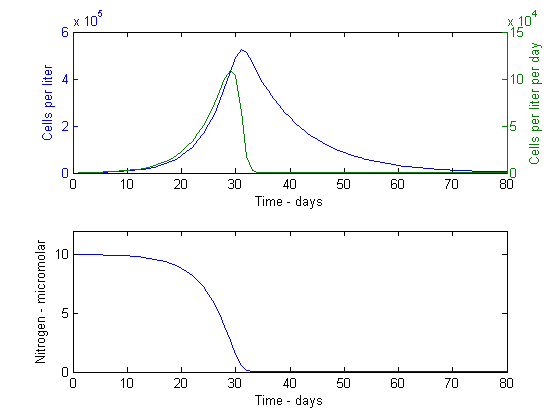# Model 2: Zooplankton class

Download the .m file for this model here.

Adapted from Frost zooplankton notes

Nutrient-limited phytoplankton growth with grazing parameters and initial conditions as Handout, part d: grazing=0.1/day=g

## Model 2: Zooplankton class

Adapted from Frost zooplankton notes

Nutrient-limited phytoplankton growth with grazing parameters and initial conditions as Handout, part d: grazing=0.1/day=g

```clear
close all
```

## Initial Conditions

```k=80;                   % 80 Duration of run, in days
t=zeros(k,1);t(1)=1;    % Initializing the time vector

P=zeros(k,1);           % Initializing the Phytoplankton vector
PHY=1000;               % 1000 Phytoplankton initial concentration- cells/L
P(1)=PHY;

PDN=zeros(k,1);         % Initializing the production vector
N=zeros(k,1);N(1)=10;   % 10 Initializing the dissolved Nitrogen vector
% Units here are in micromolar
NIT=N(1)*14;            % Converting micromolar to migrogram
% (multiply by atomic weight)

mumax=0.3465736;        % 0.3465736 Maximum phytoplankton growth rate
% Corresponds to 1 doubling per day
kn=14;                  % Half-saturation constant for nitrogen uptake
% by the phytoplankton cells
q=0.000157;             % 0.000157 Cell nigrogen content (cell quota) in
% micrograms nitrogen per cell
g=0.1;                  % 0.1 Grazing rate (per day)
```

## Main Loop

```for n=1:1:k
t(n)=n;
for m=1:1:24
% This gives an hourly timestep for the calculations and the two
% lines below recalculate the "per day" rates to "per hour"
mu=mumax*(NIT/(kn+NIT))/24;
graz=g/24;

% Phytoplankton growth and production:
kmg=mu-graz;
prod=mu*PHY*(exp(kmg)-1.0)/kmg;
PHY=PHY*exp(kmg);

% Nitrogen uptake rates:
uptake=q*prod;
NIT=NIT-uptake;

if NIT<0    % Dissolved nitrogen cannot have negative values
NIT=0;
end
end

% Here we track the results at the end of each 24 hour day
P(n)=PHY;
N(n)=NIT/14;
PDN(n)=prod*24;
end
```

## Plot the daily results

```ax1=subplot(2,1,1);
[ax,hl1,hl2]=plotyy(t,P,t,PDN);
xlabel('Time - days');
set(get(ax(1),'Ylabel'),'String','Cells per liter');
set(get(ax(2),'Ylabel'),'String','Cells per liter per day');

subplot(2,1,2)
plot(t,N)
axis([0,k,0,12]);
xlabel('Time - days');
ylabel('Nitrogen - micromolar');
```